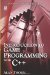# 6.12 Shorthand Operations

## 6.12 Shorthand Operations

Previous sections have shown how variables and constants can be manipulated using standard mathematical operators, such as +, , *, and /. There are, however, a number of shorthand variations that can be written to abbreviate specific expressions. Consider the operators in the following table.

Given the integer variable Num, the shorthand expressions below can be seen to mean the following:

 Num += 5 Means: Num = Num + 5 Num −= 5 Means: Num = Num −5 Num++ Means: Num = Num + 1 Num − − Means: Num = Num −1Introduction to Game Programming with C++ (Wordware Game Developers Library)
ISBN: 1598220322
EAN: 2147483647
Year: 2007
Pages: 225
Authors: Alan Thorn

Similar book on Amazon

flylib.com © 2008-2017.
If you may any questions please contact us: flylib@qtcs.net